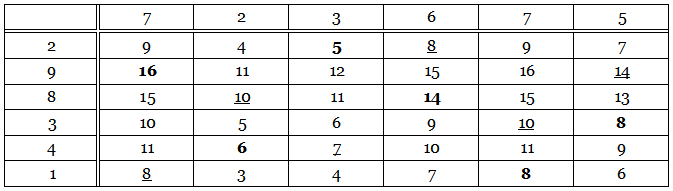# The Magic TotalEach of the 36 numbers in this table is the sum of the numbers at the head of its column and at the left of its row. For example, 3 = 2 + 1 and 13 = 5 + 8. The six bold numbers have been chosen so that each of them falls in a different row and a different column. The underlined numbers were chosen in the same way. But each of these two sextets produces the same total: 16 + 6 + 5 + 14 + 8 + 8 = 8 + 10 + 7 + 8 + 10 + 14 = 57. In fact, any six numbers chosen in this way will produce the total 57. Why is this?